Calculating Cement, Sand, and Gravel

# Calculating Cement, Sand, and Gravel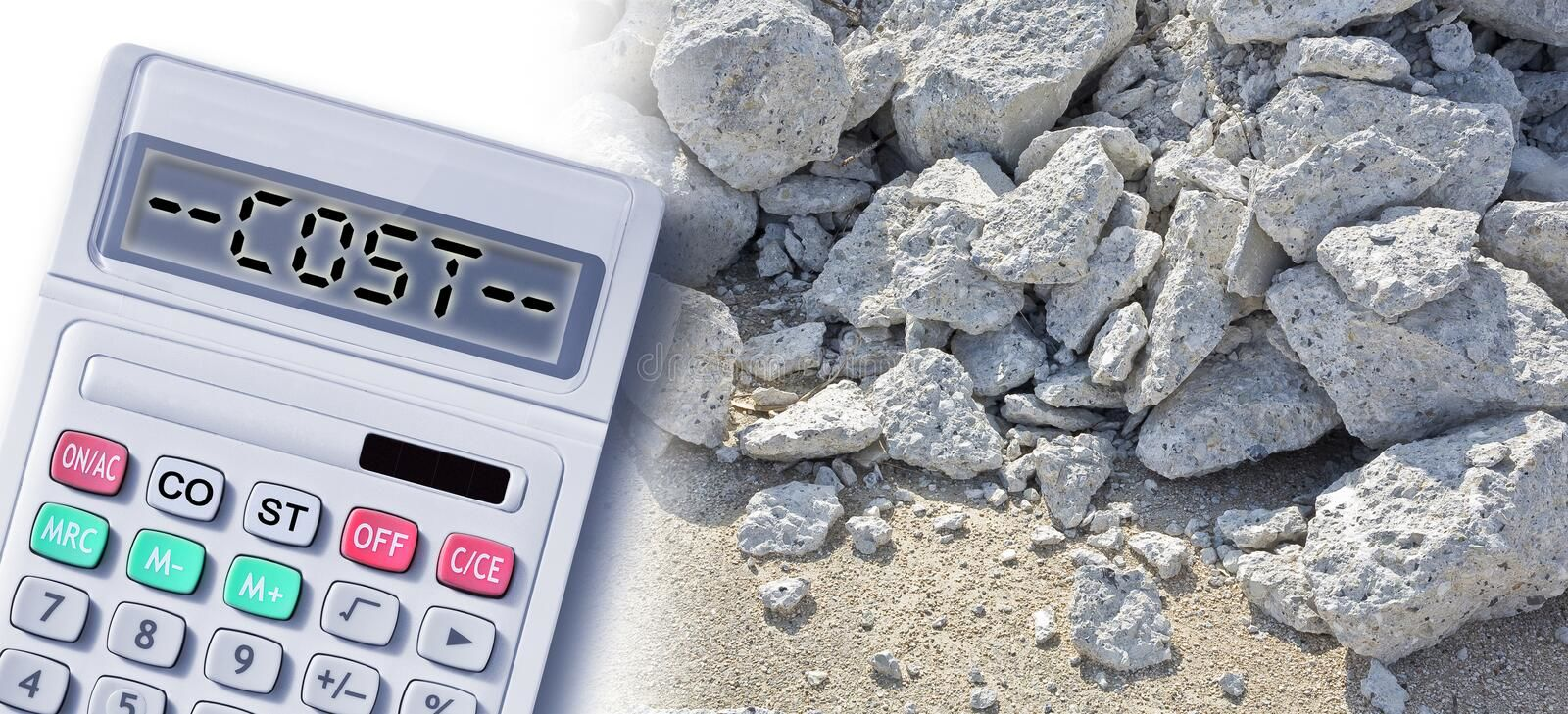The quantities of the three essential constituents (cement, sand, ang gravel) influence the physical attributes of concrete, such as density and strength. You have the option of weighing or volumetrically proportioning your ingredients. Although proportioning by volume is less exact, it may be the preferred option due to the time constraints of a class period.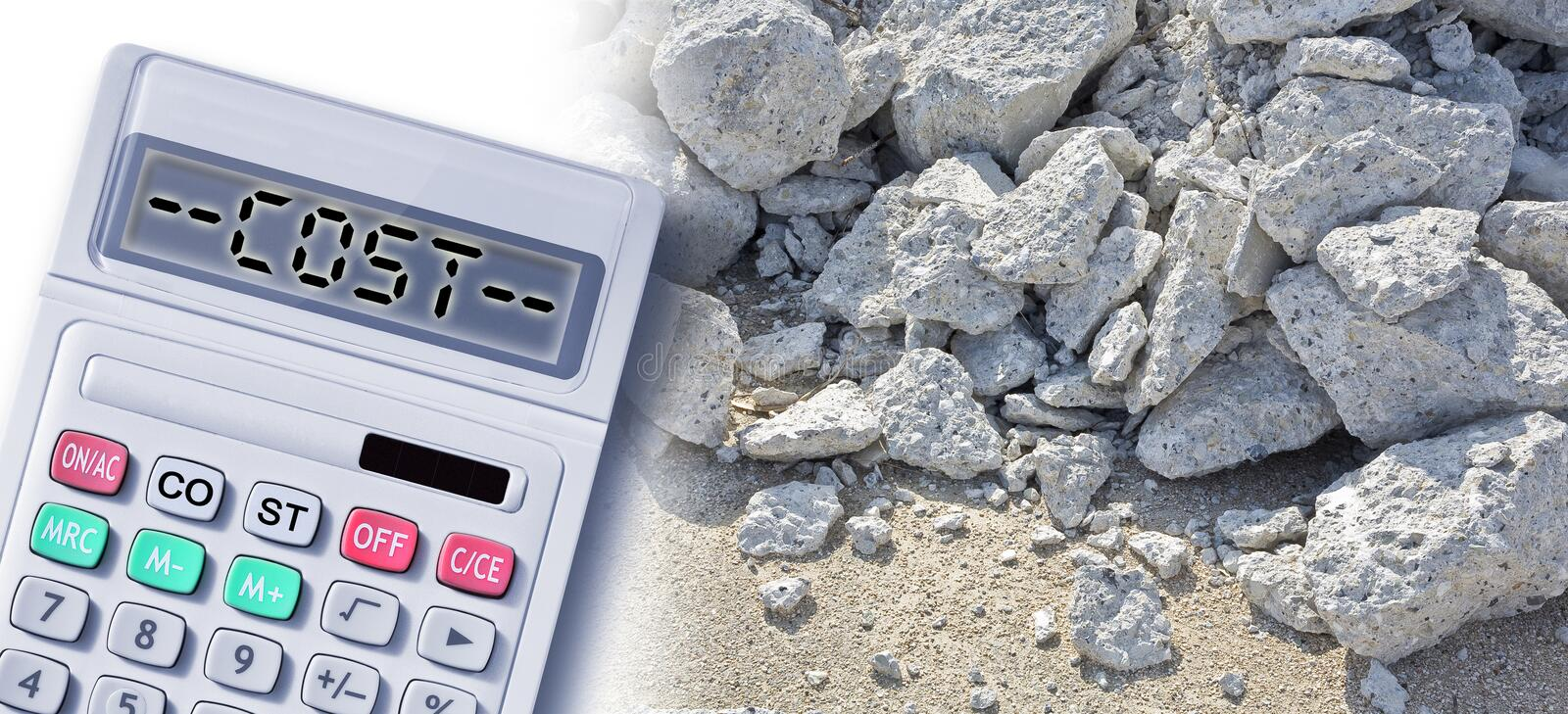Photo Courtesy: dreamstime

The volume proportions of cement with sand and gravel can be used to make a basic mortar mixture. Sand and gravel’s basic blend can be used for the majority of activities. The cement: sand: gravel by volume is another “ancient rule of thumb” for mixing concrete. Combine the dry materials in a mixing bowl and gradually add water until the concrete is pliable. Depending on the aggregate used, this mixture may need to be tweaked to provide the desired workability. It’s important that the mixture is neither too stiff nor too sloppy. If the material is overly stiff, it is difficult to make adequate test specimens. Water may separate (bleed) from the mixture if it is too sloppy.

Computing the Quantity of Cement, Sand, and Gravel for Your Concrete Mix

Cement mixture proportioning can be done either by weight or by volume. Almost all types of concrete building have traditionally used the volume method, which uses bags of cement and a measurement box as a reference for sand and gravel. Ready-mixed concrete is employed in larger and larger size projects, as well as in locations where mixing and material storage are not practical.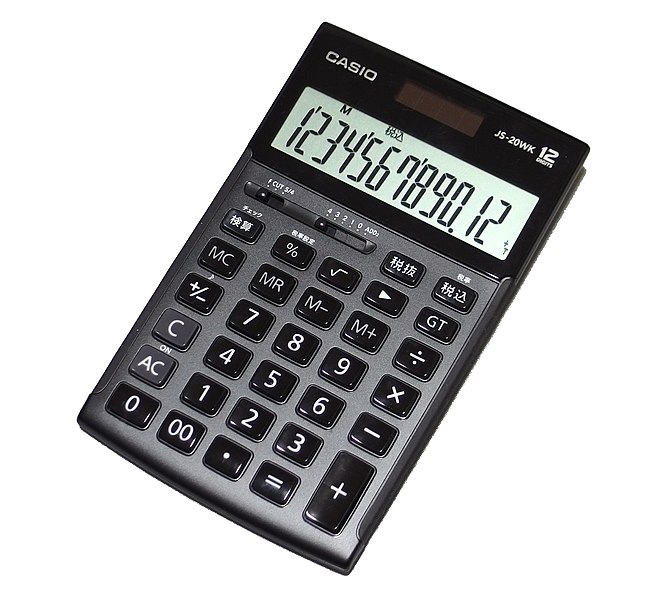Photo Courtesy: dreamstime

Points You Should Know Before Estimating

Density of cement = 1,440 kg/m3
Sand density = 1450-1500 kg/m3
Density of Aggregate = 1450-1550 kg/m3
50 kg = how many kilograms in a cement bag

In 1 cement bag, there are 34.7 liters of cement.

0.0347 cubic meter = 1 cement bag in cubic meters

1.226 Cubic Feet (CFT) equals how many Cubic Feet (CFT)?  There are 28.8 bags in a cubic meter of cement.

The specific gravity of cement is 3.15.
33, 43, and 53 are the cement grades.

Where the compressive strength of cement is 33, 43, and 53 N/mm2, respectively.

M-20 = 1:1.5:3 =5.5, M-20 = 1:1.5:3 = 5.5, M-20 = 1:1.5: (Cement : Sand : Aggregate)

Where M stands for Mix.

Characteristic compressive strength = 20
Assume a concrete volume of 1m3.

Multiply the dry volume by 1.54.

1 × 1.54 = 1.54 m3 = dry concrete volume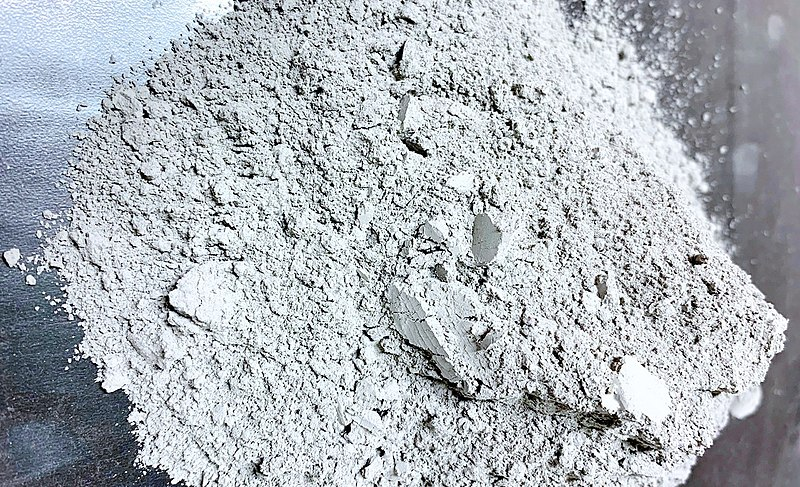Photo Courtesy: Wikimedia Commons

How to Calculate Cement, Sand, and Gravel Quantities for Your Concrete Mix

Calculate the amount of concrete you’ll need. Decide on the concrete mix class. The compressive strength of your concrete is determined by the mixture and proportions of your materials. You should choose your concrete mix class based on where the concrete will be used.

Calculate the number of bags of Portland cement you’ll need. Portland cement bags are commercially available at local hardware stores in two sizes: 40kg and 50kg. The amount of cement bags required per mix will be determined by the option you select.

Multiply your volume by the applicable values from the concrete proportion table, based on your concrete mix class and cement bag quantity.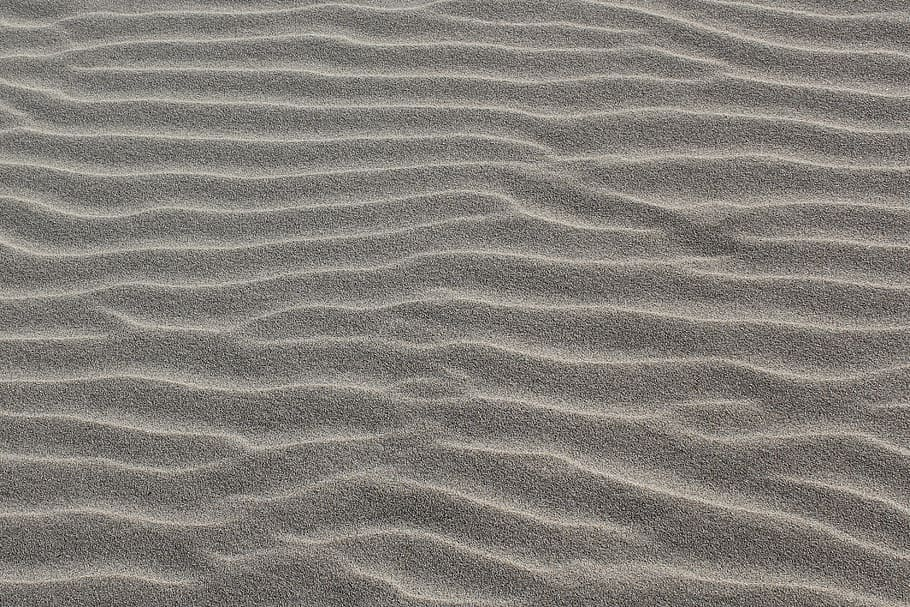Photo Courtesy: pxfuel

Sample Problem

Calculate the amount of 40kg cement bags, sand, and gravel required for a 3 meter long, 2 meter wide, and 0.150 meter thick class “A” mixture slab.

Solution

Calculate the volume of concrete mix as follows:
V = 3m * 2m * 0.150m V = 0.90m3
Volume = Length * Width * Height

Determine the concrete mix class and the number of bags of Portland cement specified:  Class “A” is the specified concrete mix class.

Determine the concrete mix class and the number of bags of Portland cement specified:  40kg specified Portland cement bag

Match and calculate sand, gravel, and cement quantities using the concrete proportion table:

Volume * Cement Multiplier for a Class “A” in 40 kg bags = 0.90m3 * 9 = 8.1 bags

The volume of sand is equal to the quantity of sand. For a Class “A” mix, the sand multiplier is 0.90m3 *.5 = 0.45m3.

Gravel Quantity equals Gravel Volume.  For a Class “A” mix, the gravel multiplier is 0.90m3 * 1 = 0.90m3.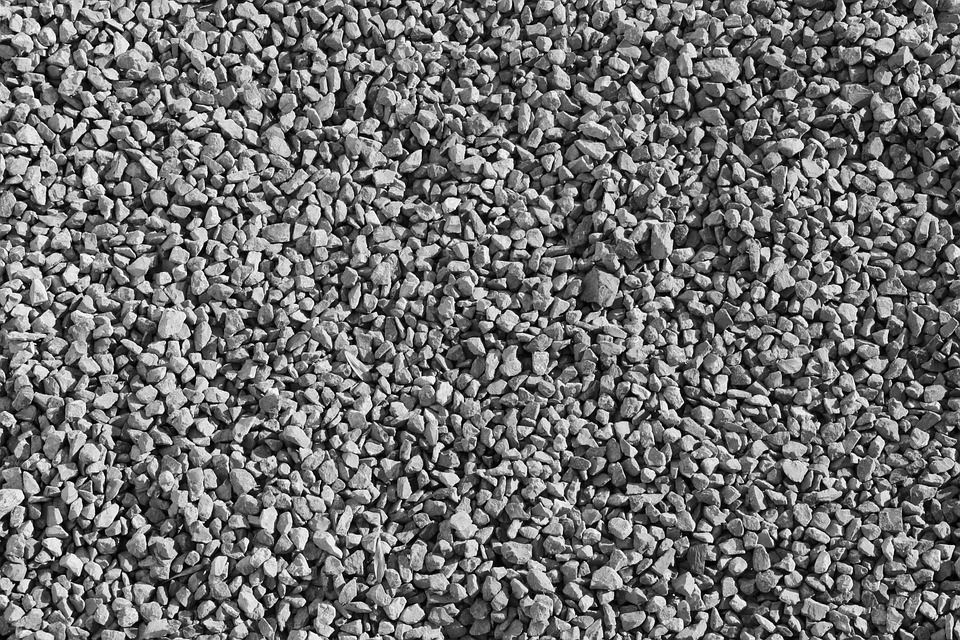Photo Courtesy: Pixabay

Sample Problem #2

Calculate the amount of 50kg cement bags, sand, and gravel required for a 5 meter long, 10 meter wide, and 0.250 meter thick class “C” mixed slab.

Solution #2

Calculate the volume of concrete mix as follows:
V = 5m * 10m * 0.250m V = 12.5m3 Volume = Length * Width * Height

Determine the concrete mix class and the number of bags of Portland cement specified:  Class “C” is the specified concrete mix class.

Determine the concrete mix class and the number of bags of Portland cement specified:  50kg specified Portland cement bag

Match and calculate sand, gravel, and cement quantities using the concrete proportion table:  Volume * Cement Multiplier for a Class “C” in 50 kg bags = 12.5m3 * 5 = 62.5 bags

The volume of sand is equal to the quantity of sand.  For a Class “C” mix, the sand multiplier is 12.5m3 *.5 = 6.25m3.

Gravel Quantity equals Gravel Volume. For a Class “C” mix, the gravel multiplier is 12.5mm3 * 1 = 12.5mm3

CITATIONS:

• “The Basic Mix: A general teacher’s guide for concrete preparation.” illinois.edu, www.matse1.matse.illinois.edu/concrete/bm.html.

• “Estimating Cement, Sand and Gravel Quantity for your Concrete Mix.” Veria,, veriaconcyclopedia.com/v/esti/esti-cm.

• “How to Calculate Cement, Sand, & Aggregate Quantity In 1 Cubic Metre Concrete” Tutorials Tips Civil, www.tutorialstipscivil.com/estimate/calculate-cement-sand-aggregate-quantity-in-1-cubic-metre-concrete.